# 线段树(单标记+离散化+扫描线+双标记)+zkw线段树+权值线段树+主席树及一些例题

“队列进出图上的方向

e.g.1（暴力膜不可取）

e.g.2（st表）

代码如下：

#include<cmath>
#include<cstdio>
#include<cstring>
#include<iostream>
#include<algorithm>
using namespace std;

int n,m,dp;

int main()
{
scanf("%d%d",&n,&m);
for(int i=1;i<=n;i++)
{
scanf("%d",&dp[i]);
}
for(int i=1;i<=17;i++)
{
for(int j=1;j<=n;j++)
{
if(j+(1<<(i-1))-1<=n)
{
dp[j][i]=min(dp[j][i-1],dp[j+(1<<(i-1))][i-1]);
}
}
}
int l,r;
for(int i=1;i<=m;i++)
{
scanf("%d%d",&l,&r);
int x=(int)(log((double)(r-l+1))/log(2.0));
printf("%d\n",min(dp[l][x],dp[r-(1<<x)+1][x]));
}
}

e.g.3（线段树点修改）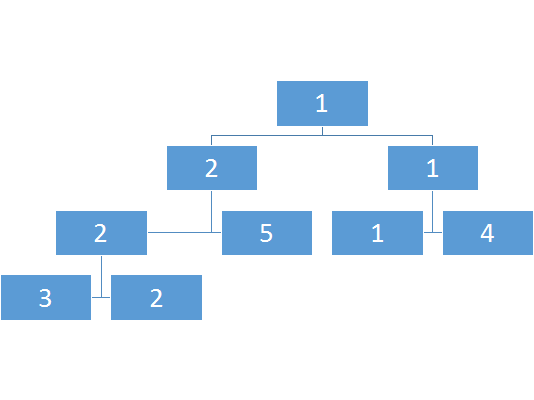区间树：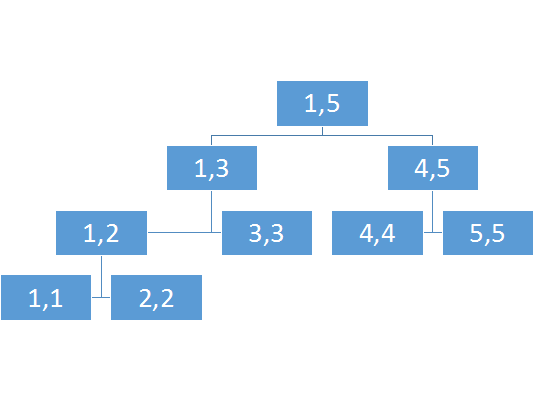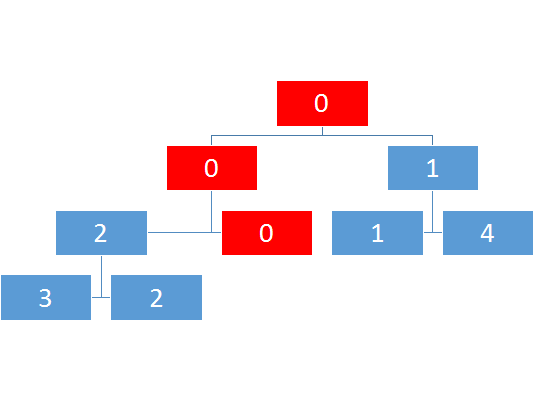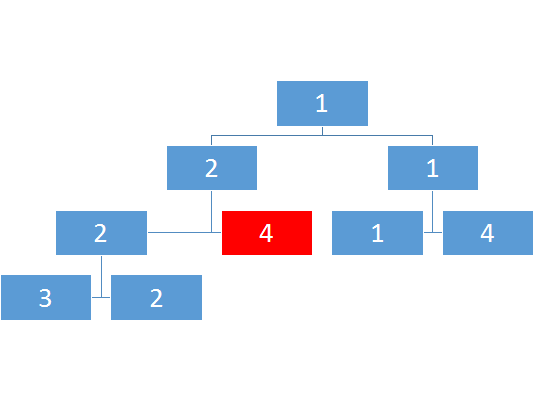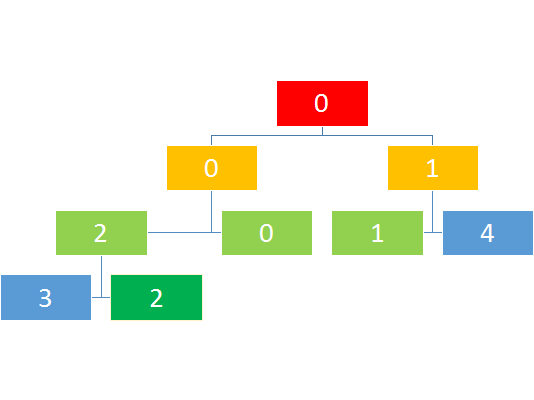e.g.3.1线段树点修改如何实现？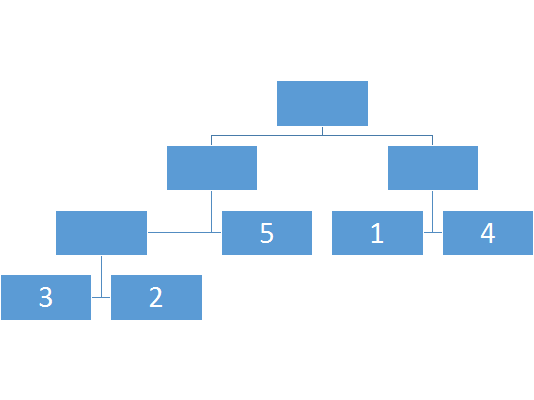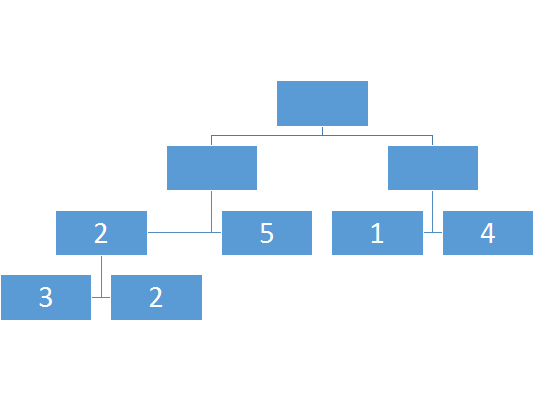1、push_up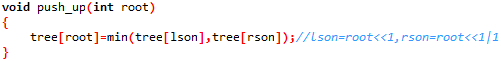2、build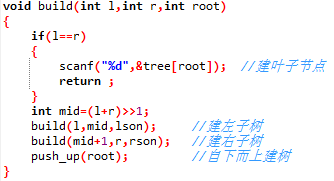update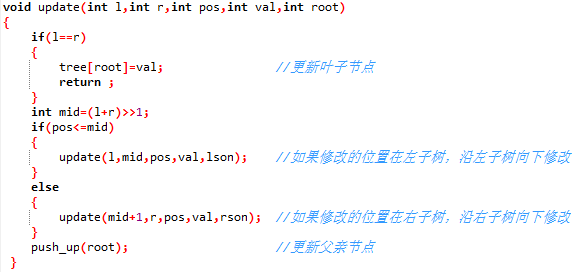query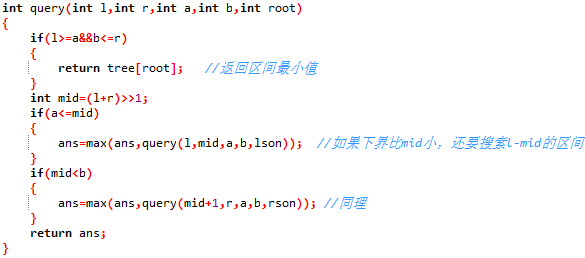hdu1754 i hate it

1、Q l r 询问l-r之间最大值

2、U x v 将x位置的值换成当前值与v值中较大的一个

5 6
1 2 3 4 5
Q 1 5
U 3 6
Q 3 4
Q 4 5
U 2 9
Q 1 5

5
6
5
9

#include<cstdio>
#include<cstring>
#include<iostream>
#include<algorithm>
using namespace std;
#define lson root<<1
#define rson root<<1|1

int tree,n,m,a,b,x,v;
char c;

void push_up(int root)
{
tree[root]=max(tree[lson],tree[rson]);
}

void build(int l,int r,int root)
{
if(l==r)
{
scanf("%d",&tree[root]);
return ;
}
int mid=(l+r)>>1;
build(l,mid,lson);
build(mid+1,r,rson);
push_up(root);
}

void update(int l,int r,int x,int v,int root)
{
if(l==r)
{
tree[root]=max(tree[root],v);
return;
}
int mid=(l+r)>>1;
if(x<=mid)
{
update(l,mid,x,v,lson);
}
else
{
update(mid+1,r,x,v,rson);
}
push_up(root);
}

int query(int a,int b,int l,int r,int root)
{
int ans=0;
if(l>=a&&b>=r)
{
return tree[root];
}
int mid=(l+r)>>1;
if(a<=mid)
{
ans=max(ans,query(a,b,l,mid,lson));
}
if(mid<b)
{
ans=max(ans,query(a,b,mid+1,r,rson));
}
return ans;
}

int main()
{
while(scanf("%d%d",&n,&m)!=EOF)
{
build(1,n,1);
for(int i=1; i<=m; i++)
{
scanf("\n%c",&c);
if(c=='Q')
{
scanf("%d%d",&a,&b);
printf("%d\n",query(a,b,1,n,1));
}
if(c=='U')
{
scanf("%d%d",&x,&v);
update(1,n,x,v,1);
}
}
}

return 0;
}

#include<cmath>
#include<cstdio>
#include<cstring>
#include<iostream>
#include<algorithm>
#define lson root<<1
#define rson root<<1|1
using namespace std;

struct node
{
int l,r,m;
} tr;

int n,m;

void push_up(int root)
{
tr[root].m=max(tr[lson].m,tr[rson].m);
}

void build(int root,int l,int r)
{
if(l==r)
{
tr[root].l=l;
tr[root].r=r;
scanf("%d",&tr[root].m);
return ;
}
tr[root].l=l;
tr[root].r=r;
int mid=(l+r)>>1;
build(lson,l,mid);
build(rson,mid+1,r);
push_up(root);
}

void update(int root,int pos,int val)
{
if(tr[root].l==pos&&tr[root].r==pos)
{
tr[root].m=max(tr[root].m,val);
return ;
}
int mid=(tr[root].l+tr[root].r)>>1;
if(pos<=mid)
{
update(lson,pos,val);
}
else
{
update(rson,pos,val);
}
push_up(root);
}

int query(int root,int l,int r)
{
if(l==tr[root].l&&r==tr[root].r)
{
return tr[root].m;
}
int mid=(tr[root].l+tr[root].r)>>1;
if(l>mid)
{
return query(rson,l,r);
}
else
{
if(mid>=r)
{
return query(lson,l,r);
}
else
{
return max(query(lson,l,mid),query(rson,mid+1,r));
}
}
}

int main()
{
while(scanf("%d%d",&n,&m)!=EOF)
{
build(1,1,n);
while(m--)
{
char kd;
scanf("\n%c",&kd);
if(kd=='Q')
{
int l,r;
scanf("%d%d",&l,&r);
printf("%d\n",query(1,l,r));
}
if(kd=='U')
{
int pos,val;
scanf("%d%d",&pos,&val);
update(1,pos,val);
}
}
}
}

hdu1166

2、Sub x v 给x的位置减v

3、Query l r 求l-r的区间和

4、End 结束询问

Sample Input
1
10
1 2 3 4 5 6 7 8 9 10
Query 1 3
Query 2 7
Sub 10 2
Query 3 10
End

Sample Output
Case 1:
6
33
59

#include<cstdio>
#include<cstring>
#include<iostream>
#include<algorithm>
using namespace std;
#define lson root<<1
#define rson root<<1|1

int tree,n,m,a,b,x,v;
char c;

void push_up(int root)
{
tree[root]=tree[lson]+tree[rson];
}

void build(int l,int r,int root)
{
if(l==r)
{
scanf("%d",&tree[root]);
return ;
}
int mid=(l+r)>>1;
build(l,mid,lson);
build(mid+1,r,rson);
push_up(root);
}

void update(int l,int r,int x,int v,int root)
{
if(l==r)
{
tree[root]+=v;
return;
}
int mid=(l+r)>>1;
if(x<=mid)
{
update(l,mid,x,v,lson);
}
else
{
update(mid+1,r,x,v,rson);
}
push_up(root);
}

int query(int a,int b,int l,int r,int root)
{
int ans=0;
if(l>=a&&b>=r)
{
return tree[root];
}
int mid=(l+r)>>1;
if(a<=mid)
{
ans+=query(a,b,l,mid,lson);
}
if(mid<b)
{
ans+=query(a,b,mid+1,r,rson);
}
return ans;
}

int main()
{
int t,ttt=0;
scanf("%d",&t);
while(t--)
{
ttt++;
printf("Case %d:\n",ttt);
memset(tree,0,sizeof(tree));
scanf("%d",&n);
build(1,n,1);
while(1)
{
cin>>c;
if(c=='Q')
{
scanf("%d%d",&a,&b);
printf("%d\n",query(a,b,1,n,1));
}
if(c=='A')
{
scanf("%d%d",&x,&v);
update(1,n,x,v,1);
}
if(c=='S')
{
scanf("%d%d",&x,&v);
update(1,n,x,-v,1);
}
if(c=='E')
{
break;
}
}
}
return 0;
}

#include<cstdio>
#include<cstring>
#include<iostream>
#include<algorithm>
#define lson root<<1
#define rson root<<1|1
using namespace std;

int gcd(int a,int b)
{
if(b>a)
{
swap(a,b);
}
if(b)
{
return gcd(b,a%b);
}
else
{
return a;
}
}

int nowson,x,cnt,n,m;

struct node
{
int l,r,g;
}tr;

void push(int root)
{
tr[root].g=gcd(tr[rson].g,tr[lson].g);
}

void build(int root,int l,int r)
{
if(l==r)
{
tr[root].l=l;
tr[root].r=r;
scanf("%d",&tr[root].g);
return ;
}
int mid=(l+r)>>1;
tr[root].l=l;
tr[root].r=r;
build(lson,l,mid);
build(rson,mid+1,r);
push(root);
}

void update(int root,int pos,int v)
{
if(tr[root].l==pos&&tr[root].r==pos)
{
tr[root].g=v;
return ;
}
int mid=(tr[root].l+tr[root].r)>>1;
if(mid>=pos)
{
update(lson,pos,v);
}
else
{
update(rson,pos,v);
}
push(root);
}

int query(int root,int l,int r)
{
if(tr[root].l==l&&tr[root].r==r)
{
if(tr[root].g%x!=0)
{
cnt--;
nowson=root;
}
return tr[root].g;
}
int mid=(tr[root].l+tr[root].r)>>1;
if(l>mid)
{
return query(rson,l,r);
}
else
{
if(r<=mid)
{
return query(lson,l,r);
}
else
{
return gcd(query(lson,l,mid),query(rson,mid+1,r));
}
}
}

int check(int root)
{
if(tr[root].l==tr[root].r)
{
return 1;
}
if(tr[lson].g%x!=0&&tr[rson].g%x!=0)
{
return 0;
}
if(tr[lson].g%x==0)
{
return check(rson);
}
else
{
return check(lson);
}
}

int main()
{
int n,m;
scanf("%d",&n);
build(1,1,n);
scanf("%d",&m);
for(int i=1;i<=m;i++)
{
int kd,l,r;
scanf("%d",&kd);
if(kd==1)
{
cnt=1;
scanf("%d%d%d",&l,&r,&x);
int tmp=query(1,l,r);
if(x==tmp)
{
puts("YES");
}
else
{
if((!cnt)&&check(nowson))
{
puts("YES");
}
else
{
puts("NO");
}
}
}
else
{
scanf("%d%d",&l,&r);
update(1,l,r);
}
}
}

e.g.4(线段树区间修改)

1.将某区间每一个数加上x（区间加

2.求出某区间每一个数的和（区间求和

#include<cstdio>
#include<vector>
#include<cstring>
#include<iostream>
#include<algorithm>
using namespace std;
#define lson root<<1
#define rson root<<1|1

long long tree;
int n,m;

void push_up(int root)
{
tree[root]=tree[lson]+tree[rson];
}

void build(int l,int r,int root)
{
if(l==r)
{
scanf("%lld",&tree[root]);
return;
}
int mid=(l+r)>>1;
build(l,mid,lson);
build(mid+1,r,rson);
push_up(root);
}

void add(int l,int r,int root,int ls,int rs,int v)
{
if(l==r)
{
tree[root]+=v;
return;
}
int mid=(l+r)>>1;
if(ls<=mid)
{
}
if(rs>mid)
{
}
push_up(root);
}

long long query(int l,int r,int ls,int rs,int root)
{
if(l>=ls&&r<=rs)
{
return tree[root];
}
int mid=(l+r)>>1;
long long ans=0;
if(ls<=mid)
{
ans+=query(l,mid,ls,rs,lson);
}
if(rs>mid)
{
ans+=query(mid+1,r,ls,rs,rson);
}
return ans;
}

int main()
{
int n,m;
scanf("%d%d",&n,&m);
build(1,n,1);
for(int i=1;i<=m;i++)
{
int kd;
int l,r,v;
scanf("%d",&kd);
if(kd==1)
{
scanf("%d%d%d",&l,&r,&v);
}
if(kd==2)
{
scanf("%d%d",&l,&r);
int ans=query(1,n,l,r,1);
printf("%lld\n",ans);
}
}
} 

#include<cstdio>
#include<vector>
#include<cstring>
#include<iostream>
#include<algorithm>
using namespace std;
#define lson root<<1
#define rson root<<1|1

long long tree;
int n,m;

void push_up(int root)
{
tree[root]=tree[lson]+tree[rson];
}

void build(int l,int r,int root)
{
if(l==r)
{
scanf("%lld",&tree[root]);
return;
}
int mid=(l+r)>>1;
build(l,mid,lson);
build(mid+1,r,rson);
push_up(root);
}

void add(int l,int r,int root,int ls,int rs,int v)
{
if(l==r)
{
tree[root]+=v;
return;
}
int mid=(l+r)>>1;
if(ls<=mid)
{
}
if(rs>mid)
{
}
push_up(root);
}

long long query(int l,int r,int ls,int rs,int root)
{
if(l>=ls&&r<=rs)
{
return tree[root];
}
int mid=(l+r)>>1;
long long ans=0;
if(ls<=mid)
{
ans+=query(l,mid,ls,rs,lson);
}
if(rs>mid)
{
ans+=query(mid+1,r,ls,rs,rson);
}
return ans;
}

int main()
{
int n,m;
scanf("%d%d",&n,&m);
build(1,n,1);
for(int i=1;i<=m;i++)
{
int kd;
int l,r,v;
scanf("%d",&kd);
if(kd==1)
{
scanf("%d%d%d",&l,&r,&v);
}
if(kd==2)
{
scanf("%d%d",&l,&r);
int ans=query(1,n,l,r,1);
printf("%lld\n",ans);
}
}
} 

ちょっとまって！我们之前的查询为什么是Θ(logN)的呢？

push_down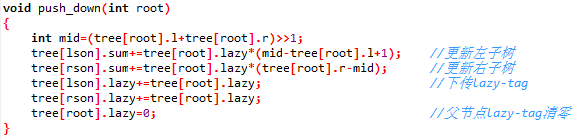#include<cstdio>
#include<cstring>
#include<iostream>
#include<algorithm>
#define lson root<<1
#define rson root<<1|1
using namespace std;

struct node
{
long long l,r,sum,lazy;
}tree;

int n,m,kd;

void push_up(int root)
{
tree[root].sum=tree[lson].sum+tree[rson].sum;
}

void push_down(int root)
{
int mid=(tree[root].l+tree[root].r)>>1;
tree[lson].sum+=tree[root].lazy*(mid-tree[root].l+1);
tree[rson].sum+=tree[root].lazy*(tree[root].r-mid);
tree[lson].lazy+=tree[root].lazy;
tree[rson].lazy+=tree[root].lazy;
tree[root].lazy=0;
}

void build(int l,int r,int root)
{
if(l==r)
{
tree[root].l=l;
tree[root].r=r;
scanf("%lld",&tree[root].sum);
return;
}
tree[root].l=l;
tree[root].r=r;
int mid=(l+r)>>1;
build(l,mid,lson);
build(mid+1,r,rson);
push_up(root);
}

void add(int l,int r,int root,int x)
{
if(l==tree[root].l&&r==tree[root].r)
{
tree[root].lazy+=x;
tree[root].sum+=x*(tree[root].r-tree[root].l+1);
return;
}
int mid=(tree[root].l+tree[root].r)>>1;
if(tree[root].lazy)
{
push_down(root);
}
if(r<=mid)
{
}
else
{
if(l>mid)
{
}
else
{
}
}
push_up(root);
}

long long query(int l,int r,int root)
{
if(l==tree[root].l&&tree[root].r==r)
{
return tree[root].sum;
}
int mid=(tree[root].l+tree[root].r)>>1;
if(tree[root].lazy)
{
push_down(root);
}
if(r<=mid)
{
return query(l,r,lson);
}
else
{
if(l>mid)
{
return query(l,r,rson);
}
}
return query(l,mid,lson)+query(mid+1,r,rson);
}

int main()
{
scanf("%d%d",&n,&m);
build(1,n,1);
for(int i=1;i<=m;i++)
{
scanf("%d",&kd);
if(kd==1)
{
int l,r,x;
scanf("%d%d%d",&l,&r,&x);
}
else
{
int l,r;
scanf("%d%d",&l,&r);
printf("%lld\n",query(l,r,1));
}
}
}

POJ2528

emmm，这道题要用离散化的思路，因为原来的区间实在是太大了！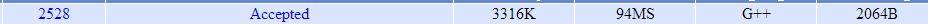#include<cmath>
#include<cstdio>
#include<cstring>
#include<iostream>
#include<algorithm>
#define lson root<<1
#define rson root<<1|1
using namespace std;

struct node
{
int l,r,val,lazy;
} tr;

struct poster
{
int l,r;
}p;

int a,cnt,ans,cnt1;

void push_down(int root)
{
tr[lson].val=tr[root].lazy;
tr[rson].val=tr[root].lazy;
tr[lson].lazy=tr[root].lazy;
tr[rson].lazy=tr[root].lazy;
tr[root].lazy=0;
}

void build(int root,int l,int r)
{
if(l==r)
{
tr[root].l=l;
tr[root].r=r;
tr[root].val=0;
return ;
}
tr[root].l=l;
tr[root].r=r;
int mid=(tr[root].l+tr[root].r)>>1;
build(lson,l,mid);
build(rson,mid+1,r);
}

void update(int root,int l,int r,int val)
{
if(l==tr[root].l&&r==tr[root].r)
{
tr[root].val=val;
tr[root].lazy=val;
return ;
}
if(tr[root].lazy)
{
push_down(root);
}
int mid=(tr[root].l+tr[root].r)>>1;
if(l>mid)
{
update(rson,l,r,val);
}
else
{
if(r<=mid)
{
update(lson,l,r,val);
}
else
{
update(lson,l,mid,val);
update(rson,mid+1,r,val);
}
}
}

int query(int root,int pos)
{
if(pos==tr[root].l&&pos==tr[root].r)
{
return tr[root].val;
}
if(tr[root].lazy)
{
push_down(root);
}
int mid=(tr[root].l+tr[root].r)>>1;
if(mid>=pos)
{
return query(lson,pos);
}
else
{
return query(rson,pos);
}
}

int main()
{
int t,n;
scanf("%d",&t);
while(t--)
{
cnt=0;
memset(cnt1,0,sizeof(cnt1));
scanf("%d",&n);
for(int i=1;i<=n;i++)
{
scanf("%d %d",&p[i].l,&p[i].r);
a[++cnt]=p[i].l;
a[++cnt]=p[i].l+1;
a[++cnt]=p[i].r;
a[++cnt]=p[i].r-1;
}
sort(a+1,a+cnt+1);               //由此开始离散化
cnt=unique(a+1,a+cnt+1)-a-1;
for(int i=1;i<=n;i++)
{
p[i].l=lower_bound(a+1,a+cnt+1,p[i].l)-a;
p[i].r=lower_bound(a+1,a+cnt+1,p[i].r)-a;    //到这结束
}
build(1,1,cnt);
for(int i=1;i<=n;i++)
{
update(1,p[i].l,p[i].r,i);
}
for(int i=1;i<=cnt;i++)
{
ans[i]=query(1,i);
}
int num=0;
for(int i=1;i<=cnt;i++)
{
if(cnt1[ans[i]]==0&&ans[i]!=0)
{
cnt1[ans[i]]=1;
num++;
}
}
printf("%d\n",num);
}
}

既然连离散化都讲了，那不妨也来讲讲扫描线吧（什么神逻辑）

e.g.乱入 扫描线

扫描线这玩意一听就非常高级啊

POJ1151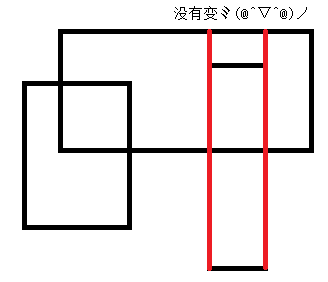push_up的改变应该很好看懂，如果这一段lazy大于0，说明区间被全覆盖，长度为区间长度，否则则是两个子树的长度之和

build建右子树的时候mid+1改成mid，建的树同时记录实数的nl-nr和伪离散化的l-r

update见代码，不是很好解释，反正不难。

#include<cstdio>
#include<cstring>
#include<iostream>
#include<algorithm>
#define lson root<<1
#define rson root<<1|1
#define N 100010
using namespace std;

struct line
{
double x,y1,y2;
int val;
} l[N];

bool cmp(line a,line b)
{
return a.x<b.x;
}

struct node
{
double nl,nr,len;
int l,r,lazy;
} tr[N<<2];

double a[N];

void push_up(int root)
{
if(tr[root].lazy>0)
{
tr[root].len=tr[root].nr-tr[root].nl;
}
else
{
if(tr[root].r-tr[root].l==1)
{
tr[root].len=0;
}
else
{
tr[root].len=tr[lson].len+tr[rson].len;
}
}
}

void build(int root,int l,int r)
{
if(l+1==r)
{
tr[root].l=l,tr[root].r=r;
tr[root].nl=a[l],tr[root].nr=a[r];
tr[root].lazy=0,tr[root].len=0.0;
return ;
}
tr[root].l=l,tr[root].r=r;
tr[root].nl=a[l],tr[root].nr=a[r];
tr[root].lazy=0,tr[root].len=0.0;
int mid=(l+r)>>1;
build(lson,l,mid);
build(rson,mid,r);
}

void update(int root,double l,double r,int val)
{
if(tr[root].nl==l&&tr[root].nr==r)
{
tr[root].lazy+=val;
push_up(root);
return ;
}
if(r<=tr[lson].nr)
{
update(lson,l,r,val);
}
else
{
if(l>=tr[rson].nl)
{
update(rson,l,r,val);
}
else
{
update(lson,l,tr[lson].nr,val);
update(rson,tr[rson].nl,r,val);
}
}
push_up(root);
}

int main()
{
int n,cnt,ttt=0;
while(scanf("%d",&n)!=EOF&&n)
{
ttt++;
cnt=0;
double x1,x2,y1,y2;
for(int i=1;i<=n;i++)
{
scanf("%lf%lf%lf%lf",&x1,&y1,&x2,&y2);
l[++cnt].x=x1;l[cnt].y1=y1;l[cnt].y2=y2;l[cnt].val=1;
a[cnt]=y1;
l[++cnt].x=x2;l[cnt].y1=y1;l[cnt].y2=y2;l[cnt].val=-1;
a[cnt]=y2;
}
sort(l+1,l+cnt+1,cmp);
sort(a+1,a+cnt+1);
build(1,1,cnt);
update(1,l.y1,l.y2,l.val);
double ans=0.0;
for(int i=2;i<=cnt;i++)
{
ans+=tr.len*(l[i].x-l[i-1].x);
update(1,l[i].y1,l[i].y2,l[i].val);
}
printf("Test case #%d\n",ttt);
printf("Total explored area: %.6lf\n",ans);
puts("");
}
}

e.g.5(线段树区间修改——双标记)

啊，你终于帮助老师写出了一颗带区间修改的线段树，结果老师眉头一皱，发现事情并不简单。

(a+(b/c))*c

emmm,看，我的精度，他飞起来啦qwq

sorry，先乘再加是真的可以为所欲为的！

a*c+b

a*c+b*c

1.将某区间每一个数乘上x

2.将某区间每一个数加上x

3.求出某区间每一个数的和

#include<cstdio>
#include<cstring>
#include<iostream>
#include<algorithm>
#define ll long long
#define lson root<<1
#define rson root<<1|1
#define N 400010
using namespace std;

struct node
{
}tree[N];

int n,m,p;

void push_up(int root)
{
tree[root].sum=(tree[lson].sum+tree[rson].sum)%p;
}

void push_down(int root)
{
int mid=(tree[root].l+tree[root].r)>>1;
tree[lson].mul=(tree[lson].mul*tree[root].mul)%p;
tree[rson].mul=(tree[rson].mul*tree[root].mul)%p;
tree[root].mul=1;
return;
}

void build(int l,int r,int root)
{
if(l==r)
{
tree[root].l=l;
tree[root].r=r;
scanf("%lld",&tree[root].sum);
return;
}
tree[root].l=l;
tree[root].r=r;
int mid=(l+r)>>1;
build(l,mid,lson);
build(mid+1,r,rson);
push_up(root);
return;
}

void add(int l,int r,int root,ll k)
{
if(l==tree[root].l&&r==tree[root].r)
{
tree[root].sum=(tree[root].sum+k*(tree[root].r-tree[root].l+1))%p;
return ;
}
int mid=(tree[root].l+tree[root].r)>>1;
push_down(root);
if(r<=mid)
{
}
else
{
if(l>mid)
{
}
else
{
}
}
push_up(root);
}

void mul(int l,int r,int root,ll k)
{
if(l==tree[root].l&&r==tree[root].r)
{
tree[root].sum=(tree[root].sum*k)%p;
tree[root].mul=(tree[root].mul*k)%p;
return ;
}
int mid=(tree[root].l+tree[root].r)>>1;
push_down(root);
if (r<=mid)
{
mul(l,r,lson,k);
}
else
{
if (l>mid)
{
mul(l,r,rson,k);
}
else
{
mul (l,mid,lson,k);
mul(mid+1,r,rson,k);
}
}
push_up(root);
}

ll query (int l,int r,int root)
{
if(l==tree[root].l&&r==tree[root].r)
{
return tree[root].sum%p;
}
int mid=(tree[root].l+tree[root].r)>>1;
push_down(root);
if(r<=mid)
{
return query(l,r,lson);
}
else
{
if(l>mid)
{
return query(l,r,rson);
}
else
{
return (query(l,mid,lson)+query(mid+1,r,rson))%p;
}
}
}

int main ()
{
scanf("%d%d%d",&n,&m,&p);
build(1,n,1);
for(int i=1; i<=m; ++i)
{
int oper;
scanf("%d",&oper);
if(oper==1)
{
int l,r;
ll k;
scanf("%d%d%lld",&l,&r,&k);
mul(l,r,1,k);
}
else
{
if(oper==2)
{
int l,r;
ll k;
scanf("%d%d%lld",&l,&r,&k);
}
else
{
int l,r;
scanf("%d%d",&l,&r);
printf("%lld\n",query(l,r,1)%p);
}
}
}

return 0;
}

e.g.6(zkw线段树点修改)

zkw线段树又被称为非递归版线段树，常数比普通线段树要小很多，这是因为zkw线段树用的几乎都是for循环，这省去了很多时间和空间。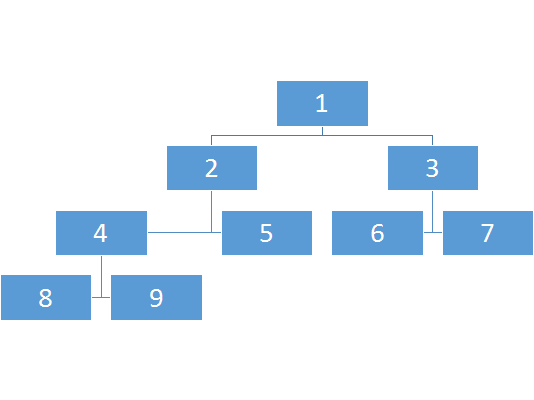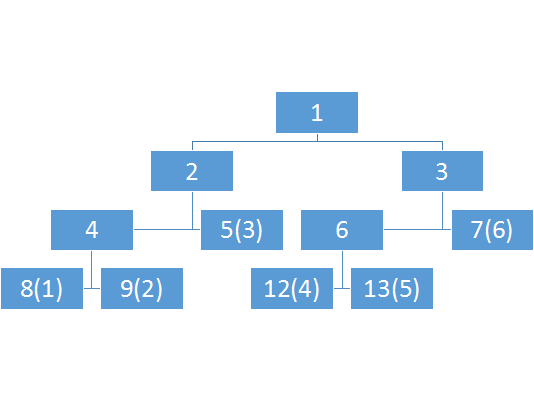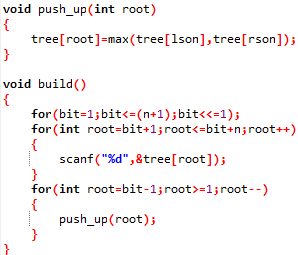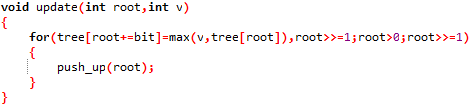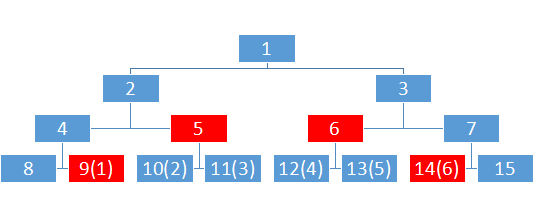naive！

来来来，再看看上面这张图

11的上面是5,，恭喜你，顺便把2也算进去了

能够完成logn的查询区间最大值，而且不会错误的将不属于该区间的块记录进来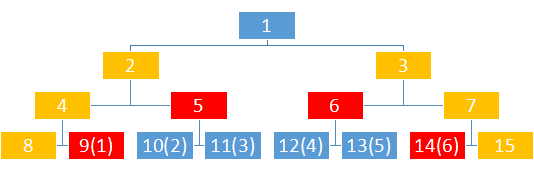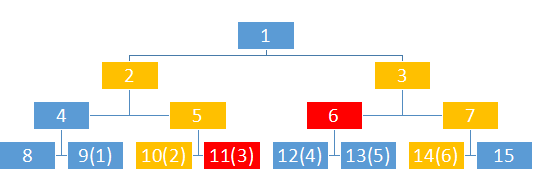#include<cstdio>
#include<cstring>
#include<iostream>
#include<algorithm>
using namespace std;
#define lson root<<1
#define rson root<<1|1

int n,m,bit;
char c;
int tree;

void push_up(int root)
{
tree[root]=max(tree[lson],tree[rson]);
}

void build()
{
for(bit=1; bit<=(n+1); bit<<=1);
for(int root=bit+1; root<=bit+n; root++)
{
scanf("%d",&tree[root]);
}
for(int root=bit-1; root>=1; root--)
{
push_up(root);
}
}

void update(int root,int v)
{
for(tree[root+=bit]=v,root>>=1; root>0; root>>=1)
{
push_up(root);
}
}

int query(int l,int r)
{
int ans=0;
for(l+=bit-1,r+=bit+1; l^r^1; l>>=1,r>>=1)
{
if(~l&1)
{
ans=max(ans,tree[l^1]);
}
if(r&1)
{
ans=max(ans,tree[r^1]);
}
}
return ans;
}

int main()
{
while(scanf("%d%d",&n,&m)!=EOF)
{
build();
for(int i=1; i<=m; i++)
{
int a,b;
scanf("\n%c%d%d",&c,&a,&b);
if(c=='Q')
{
printf("%d\n",query(a,b));
}
else
{
update(a,b);
}
}
}
}

zkw线段树单点修改区间求和也是好写的， 以hdu1166为例

#include<cstdio>
#include<cstring>
#include<iostream>
#include<algorithm>
using namespace std;
#define lson root<<1
#define rson root<<1|1

int n,m,bit;
char c;
int tree;

void push_up(int root)
{
tree[root]=tree[lson]+tree[rson];
}

void build()
{
for(bit=1; bit<=(n+1); bit<<=1);
for(int root=bit+1; root<=bit+n; root++)
{
scanf("%d",&tree[root]);
}
for(int root=bit-1; root>=1; root--)
{
push_up(root);
}
}

void update(int root,int v)
{
for(tree[root+=bit]+=v,root>>=1; root>0; root>>=1)
{
push_up(root);
}
}

int query(int l,int r)
{
int ans=0;
for(l+=bit-1,r+=bit+1; l^r^1; l>>=1,r>>=1)
{
if(~l&1)
{
ans+=tree[l^1];
}
if(r&1)
{
ans+=tree[r^1];
}
}
return ans;
}

int main()
{
int t,ttt=0;
scanf("%d",&t);
while(t--)
{
ttt++;
printf("Case %d:\n",ttt);
memset(tree,0,sizeof(tree));
scanf("%d",&n);
build();
for(; 1; )
{
int a,b;
scanf("\n%s",&c);
if(c=='E')
{
break;
}
scanf("%d %d",&a,&b);
if(c=='Q')
{
printf("%d\n",query(a,b));
}
else
{
if(c=='A')
{
update(a,b);
}
else
{
if(c=='S')
{
update(a,-b);
}
}
}
}
}
}

至于zkw线段树有多优越，看看下面这两张图就知道了~感谢xhk提供的大常数普通线段树~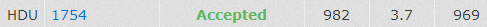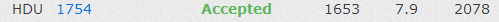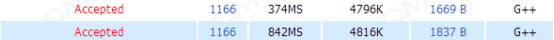e.g.7(权值线段树求第k小，rank，前驱后继)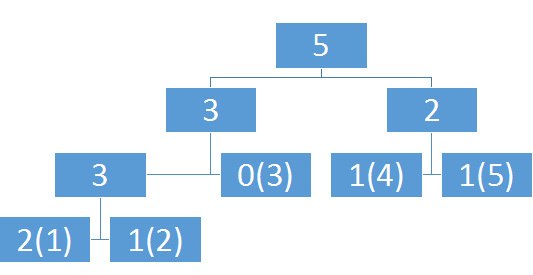1. 插入x
2. 删除x
3. 查询x
4. 查询排名为x
5. x
6. x

emmm，n还是可以接受的，但是值域……太大了啊！

kth和排名都可以logn搞，前驱后继我瞎胡了一种log^2 n的做法，如果有大佬能够给出logn的做法还请不吝赐教~

#include<cstdio>
#include<cstring>
#include<iostream>
#include<algorithm>
#define lson root<<1
#define rson root<<1|1
using namespace std;

struct node
{
int l,r,sum;
} tr;

struct opt
{
int kd,x;
} op;

int cnt,a;

void push_up(int root)
{
tr[root].sum=tr[lson].sum+tr[rson].sum;
}

void build(int root,int l,int r)
{
if(l==r)
{
tr[root].l=l;
tr[root].r=r;
tr[root].sum=0;
return ;
}
tr[root].l=l;
tr[root].r=r;
int mid=(l+r)>>1;
build(lson,l,mid);
build(rson,mid+1,r);
push_up(root);
}

void update(int root,int pos,int val)
{
if(tr[root].l==pos&&tr[root].r==pos)
{
tr[root].sum+=val;
return ;
}
int mid=(tr[root].l+tr[root].r)>>1;
if(mid>=pos)
{
update(lson,pos,val);
}
else
{
update(rson,pos,val);
}
push_up(root);
}

int query(int root,int l,int r)
{
if(l>r)
{
return 0;
}
if(tr[root].l==l&&tr[root].r==r)
{
return tr[root].sum;
}
int mid=(tr[root].l+tr[root].r)>>1;
if(mid<l)
{
return query(rson,l,r);
}
else
{
if(r<=mid)
{
return query(lson,l,r);
}
else
{
return query(lson,l,mid)+query(rson,mid+1,r);
}
}
}

int kth(int root,int k)
{
if(tr[root].l==tr[root].r)
{
return tr[root].l;
}
if(tr[lson].sum>=k)
{
return kth(lson,k);
}
else
{
return kth(rson,k-tr[lson].sum);
}
}

int rank(int x)
{
int pos=lower_bound(a+1,a+cnt+1,x)-a;
return query(1,1,pos-1)+1;
}

int pre(int root,int pos)
{
int l=1,r=pos-1,mid;
while(l<r)
{
mid=(l+r)>>1;
if(query(1,mid+1,r))
{
l=mid+1;
}
else
{
r=mid;
}
}
return r;
}

int next(int root,int pos)
{
int l=pos+1,r=cnt,mid;
while(l<r)
{
mid=(l+r)>>1;
if(query(1,l,mid))
{
r=mid;
}
else
{
l=mid+1;
}
}
return l;
}

int main()
{
int n;
scanf("%d",&n);
for(int i=1; i<=n; i++)
{
scanf("%d%d",&op[i].kd,&op[i].x);
if(op[i].kd!=2&&op[i].kd!=4)
{
a[++cnt]=op[i].x;
}
}
sort(a+1,a+cnt+1);
cnt=unique(a+1,a+cnt+1)-a-1;
build(1,1,cnt);
for(int i=1; i<=n; i++)
{
if(op[i].kd==1)
{
int pos=lower_bound(a+1,a+cnt+1,op[i].x)-a;
update(1,pos,1);
}
if(op[i].kd==2)
{
int pos=lower_bound(a+1,a+cnt+1,op[i].x)-a;
update(1,pos,-1);
}
if(op[i].kd==3)
{
printf("%d\n",rank(op[i].x));
}
if(op[i].kd==4)
{
int pos=kth(1,op[i].x);
printf("%d\n",a[pos]);
}
if(op[i].kd==5)
{
int pos=lower_bound(a+1,a+cnt+1,op[i].x)-a;
printf("%d\n",a[pre(1,pos)]);
}
if(op[i].kd==6)
{
int pos=lower_bound(a+1,a+cnt+1,op[i].x)-a;
printf("%d\n",a[next(1,pos)]);
}
}
}

e.g.8（主席树求区间第k小）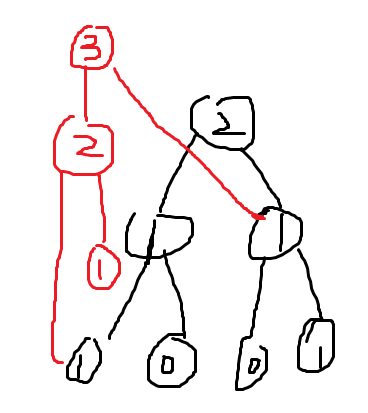#include<cstdio>
#include<cstring>
#include<iostream>
#include<algorithm>
#define mid ((l+r)>>1)
#define hi puts("hi");
using namespace std;
#define N 200010

int n,m,q,cnt=0;
int a[N],b[N],T[N];
int sum[N<<5],L[N<<5],R[N<<5];

int build(int l,int r)
{
int rt=++cnt;
sum[rt]=0;
if(l<r)
{
L[rt]=build(l,mid);
R[rt]=build(mid+1,r);
}
return rt;
}

int update(int pre,int l,int r,int x)
{
int rt=++cnt;
L[rt]=L[pre];
R[rt]=R[pre];
sum[rt]=sum[pre]+1;
if(l<r)
{
if(x<=mid)
{
L[rt]=update(L[pre],l,mid,x);
}
else
{
R[rt]=update(R[pre],mid+1,r,x);
}
}
return rt;
}

int query(int u,int v,int l,int r,int k)
{
if(l>=r)
{
return l;
}
int x=sum[L[v]]-sum[L[u]];
if(x>=k)
{
return query(L[u],L[v],l,mid,k);
}
else
{
return query(R[u],R[v],mid+1,r,k-x);
}
}

int main()
{
scanf("%d%d",&n,&q);
for(int i=1;i<=n;i++)
{
scanf("%d",&a[i]);
b[i]=a[i];
}
sort(b+1,b+n+1);
m=unique(b+1,b+1+n)-b-1;
T=build(1,m);
for(int i=1;i<=n;i++)
{
int t=lower_bound(b+1,b+1+m,a[i])-b;
T[i]=update(T[i-1],1,m,t);
}
while(q--)
{
int ll,rr,kk;
scanf("%d%d%d",&ll,&rr,&kk);
int t=query(T[ll-1],T[rr],1,m,kk);
printf("%d\n",b[t]);
}
return 0;
}

*均一天3000字（不含制图）真的快要赶上网文作家了，代码写到死啊，不过还是很有成就感的

posted @ 2018-04-17 19:17  Styx-ferryman  阅读(6593)  评论(4编辑  收藏  举报